Home Practice
For learners and parents For teachers and schools
Textbooks
Full catalogue
Pricing SupportLog in

We think you are located in United States. Is this correct?

# 13.2 Right prisms and cylinders

## 13.2 Right prisms and cylinders (EMA7M)

Right prism

A right prism is a geometric solid that has a polygon as its base and vertical faces perpendicular to the base. The base and top surface are the same shape and size. It is called a “right” prism because the angles between the base and faces are right angles.

A triangular prism has a triangle as its base, a rectangular prism has a rectangle as its base, and a cube is a rectangular prism with all its sides of equal length. A cylinder has a circle as its base. Examples of right prisms and a cylinder are given below: a rectangular prism, a cube and a triangular prism.

### Surface area of prisms and cylinders (EMA7N)

Surface area

Surface area is the total area of the exposed or outer surfaces of a prism.

This is easier to understand if we imagine the prism to be a cardboard box that we can unfold. A solid that is unfolded like this is called a net. When a prism is unfolded into a net, we can clearly see each of its faces. In order to calculate the surface area of the prism, we can then simply calculate the area of each face, and add them all together.

For example, when a triangular prism is unfolded into a net, we can see that it has two faces that are triangles and three faces that are rectangles. To calculate the surface area of the prism, we find the area of each triangle and each rectangle, and add them together.

In the case of a cylinder the top and bottom faces are circles and the curved surface flattens into a rectangle with a length that is equal to the circumference of the circular base. To calculate the surface area we therefore find the area of the two circles and the rectangle and add them together.

Below are examples of right prisms and a cylinder that have been unfolded into nets:

Rectangular prism

A rectangular prism unfolded into a net is made up of six rectangles.

Cube

A cube unfolded into a net is made up of six identical squares.

Triangular prism

A triangular prism unfolded into a net is made up of two triangles and three rectangles. The sum of the lengths of the rectangles is equal to the perimeter of the triangles.

Cylinder

A cylinder unfolded into a net is made up of two identical circles and a rectangle with a length equal to the circumference of the circles.

This video explains how we can unfold solids into nets.

Video: 2GS2

It may be useful to have some nets of the different polyhedra available for learners to see how they fold up to form the polyhedra.

You can download and print out nets of various polyhedra from senteacher to use in your classroom.

## Worked example 2: Finding the surface area of a rectangular prism

Find the surface area of the following rectangular prism: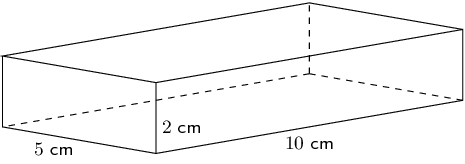### Sketch and label the net of the prism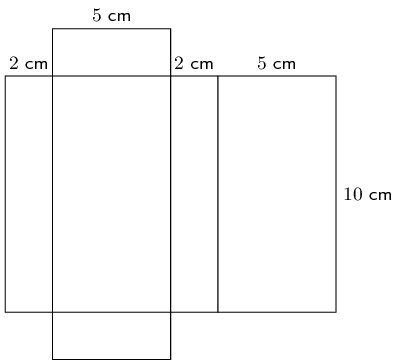### Find the areas of the different shapes in the net

\begin{align*} \text{large rectangle}& =\text{perimeter of small rectangle}\times \text{length} \\ & = \left(2 + 5 + 2 + 5\right)\times 10 \\ & = 14\times 10 \\ & = \text{140}\text{ cm$^{2}$} \\ \\ 2\times \text{small rectangle}& = 2\left(5\times 2\right) \\ & = 2\left(10\right) \\ & = \text{20}\text{ cm$^{2}$} \end{align*}

### Find the sum of the areas of the faces

$$\text{large rectangle} + 2\times \text{small rectangle} = 140 + 20 = 160$$

The surface area of the rectangular prism is $$\text{160}$$ $$\text{cm^{2}}$$.

temp text

## Worked example 3: Finding the surface area of a triangular prism

Find the surface area of the following triangular prism: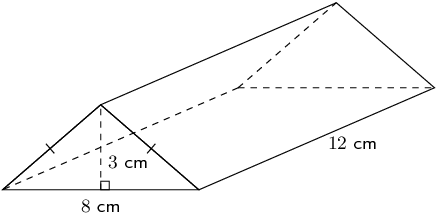### Sketch and label the net of the prism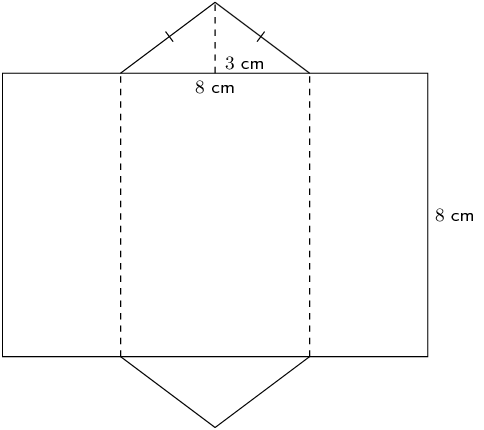### Find the area of the different shapes in the net

To find the area of the rectangle, we need to calculate its length, which is equal to the perimeter of the triangles.

To find the perimeter of the triangle, we have to first find the length of its sides using the theorem of Pythagoras: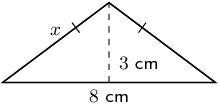### Find the sum of the areas of the faces

\begin{align*} \text{surface area}& = \text{area large rectangle} + \left(2\times \text{area of triangle}\right) \\ & = 216 + 2\left(12\right) \\ & = \text{240}\text{ cm$^{2}$} \end{align*}

The surface area of the triangular prism is $$\text{240}$$ $$\text{cm^{2}}$$.

temp text

## Worked example 4: Finding the surface area of a cylinder

Find the surface area of the following cylinder (correct to 1 decimal place):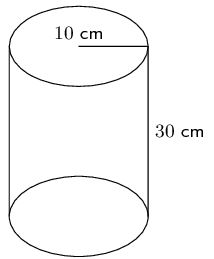### Sketch and label the net of the cylinder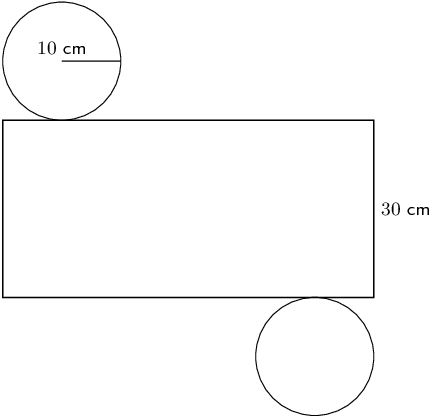### Find the area of the different shapes in the net

\begin{align*} \text{area of large rectangle} & = \text{circumference of circle}\times \text{length} \\ & = 2 \pi r \times l \\ & = 2 \pi \left(10\right)\times 30 \\ & = \text{1 884,9555...}\text{ cm$^{2}$} \\ \text{area of circle} & = \pi {r}^{2} \\ & = \pi {(10)}^{2} \\ & = \text{314,1592...}\text{ cm$^{2}$} \end{align*}\begin{align*} \text{surface area}& =\text{area large rectangle}+\left(2\times \text{area of circle}\right) \\ & =\text{1 884,9555...} + 2\left(\text{314,1592...}\right) \\ & = \text{2 513,3}\text{ cm$^{2}$} \end{align*}

The surface area of the cylinder is $$\text{2 513,3}$$ $$\text{cm^{2}}$$.

temp text
Textbook Exercise 13.2

Calculate the surface area of the following prisms: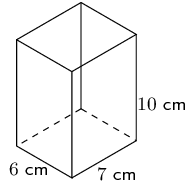\begin{align*} \text{Area of large rectangle} &= \text{perimeter of small rectangle} \times \text{length} \\ &= (10 + 7 + 10 + 7) \times 6 \\ &= 34 \times 6 \\ &= \text{204}\text{ cm$^{2}$} \\ \text{Area of } 2 \times \text{ small rectangle} &= 2(7 \times 10) \\ &= 2(70) \\ &= \text{140}\text{ cm$^{2}$} \\ \text{Area of large rectangle} + 2 \times \text{(small rectangle)} &= 204 + 140 = \text{344}\text{ cm$^{2}$} \end{align*}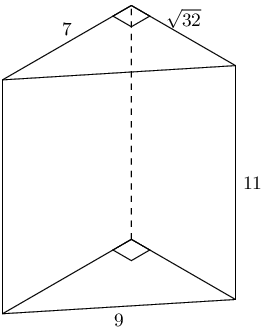There are three different sized rectangles that make up the sides of this triangular prism. We need to find the area of each one of them. All of the rectangles have a height of 11 but each rectangle has a different base.

\begin{align*} \text{area of } 2 \times \text{ triangle} &= 2\left(\frac{1}{2}b \times h\right) \\ &= (\sqrt{32})(7) \\ & = \text{39,5979...} \\ \text{area of rectangle 1} &= b \times h \\ &= (7)(11) \\ & = 77 \\ \text{area of rectangle 2} &= b \times h \\ &= (9)(11) \\ & = 99 \\ \text{area of rectangle 3} &= b \times h \\ &= (\sqrt{32})(11) \\ & = \text{62,2253...} \\ A_{\text{triangular prism}} & = \text{39,5979...} + 77 + 99 + \text{62,2253...} \\ & = \text{277,82}\text{ cm$^{2}$} \end{align*}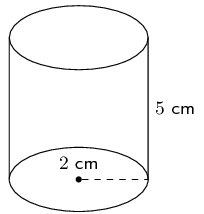\begin{align*} \text{Area of large rectangle} &= \text{circumference of circle} \times \text{length}\\ &= 2 \pi \times r \times l \\ &= 2 \pi \times (2) \times 5 \\ &= 20 \pi \\ \text{Area of circle}&= \pi r^{2}\\ &= \pi (2)^{2}\\ &= 4 \pi \\ \text{Surface area} &= \text{area large rectangle} + 2(\text{area of circle})\\ & = 20 \pi + 2(4 \pi)\\ & = 28 \pi \\ & \approx \text{87,96}\text{ cm$^{2}$} \end{align*}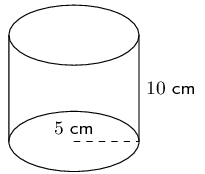\begin{align*} \text{Area of large rectangle} &= \text{circumference of circle} \times \text{length}\\ &= 2 \pi \times r \times l \\ &= 2 \pi \times (5) \times 10 \\ &= 100 \pi \\ \text{Area of circle}&= \pi r^{2}\\ &= \pi (5)^{2}\\ &= 25 \pi \\ \text{Surface area} &= \text{area large rectangle} + 2(\text{area of circle})\\ & = 100 \pi + 2(25 \pi)\\ & = 150 \pi \\ & \approx \text{471,24}\text{ cm$^{2}$} \end{align*}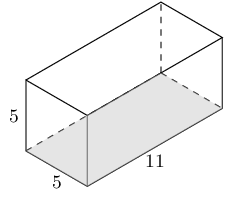There are 4 rectangles and 2 squares that make up this rectangular prism. The square has a side length of 5. The rectangles have a base of 5 and a height of 11.

\begin{align*} A_{\text{rectangular prism}} & = 4 \times \text{area rectangle} + 2 \times \text{area square} \\ & = 4(b \times h) + 2(s^{2}) \\ & = 4(11 \times 5) + 2(5^{2}) \\ & = 4(55) + 2(25) \\ & = \text{270} \end{align*}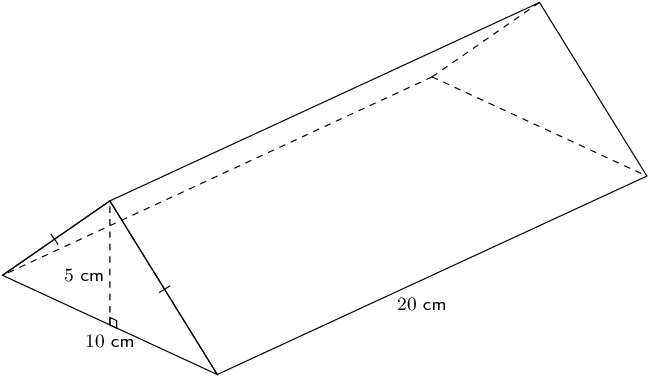We first need to find the missing side of the triangle. We can do this using the theorem of Pythagoras.

\begin{align*} x^2 &= 5^2 + \left(\frac{10}{2}\right)^2\\ x^2& = 5^2 + 5^2 \\ & = 25 + 25 \\ x &= \sqrt{50} \end{align*}

Now we can find the area of the triangular prism:

\begin{align*} \text{perimeter of triangle} &= 10 + \sqrt{50} + \sqrt{50} \\ &= \text{24,1421...} \\ \text{area of large rectangle} &= \text{perimeter of triangle} \times \text{length}\\ &= \text{24,1421...} \times 20 \\ & = \text{482,8427...} \\ \text{area of triangle} &= \frac{1}{2} b \times h \\ &= \frac{1}{2} \times 5 \times 10 \\ &= \text{25} \\ \text{surface area} &= \text{area large rectangle} + 2(\text{area of triangle})\\ &= \text{482,8427...} + 2(25)\\ &= \text{532,84}\text{ cm$^{2}$} \end{align*}

If a litre of paint covers an area of $$\text{2}$$ $$\text{m^{2}}$$, how much paint does a painter need to cover:

a rectangular swimming pool with dimensions $$\text{4}\text{ m} \times \text{3}\text{ m} \times \text{2,5}\text{ m}$$ (the inside walls and floor only);

We need to find the surface area of the pool. In this case we have a rectangular prism but with one rectangle missing (which would be the top of the pool).

\begin{align*} \text{surface area} &= \text{area of bottom of pool} + 2\text{(area of long sides)}\\ & + 2\text{(area of short sides)}\\ &= (4 \times 3) + 2(4 \times \text{2,5}) + 2(3 \times \text{2,5})\\ &= 12 + 20 + 15\\ &= \text{47}\text{ m$^{2}$} \end{align*}

The painter needs one litre of paint for every $$\text{2}$$ $$\text{m^{2}}$$ of area. So we must divide the surface area by 2 to find the total amount of paint needed. Therefore, the painter will need $$\frac{47}{2} = \text{24}\text{ l}$$ of paint (rounded up to the nearest litre).

the inside walls and floor of a circular reservoir with diameter $$\text{4}$$ $$\text{m}$$ and height $$\text{2,5}$$ $$\text{m}$$.

We need to find the surface area of the reservoir. In this case we have a cylinder but with one circle missing (which would be the top of the reservoir).

We are given the diameter of the reservoir. The radius is half the diameter and so $$r = \text{2}\text{ m}$$.

\begin{align*} \text{surface area} &= \text{area of bottom of reservoir} + \text{area of inside of reservoir}\\ & = (\pi r^2) + (\text{circumference of base} \times \text{height of reservoir})\\ & = (\pi (2)^2) + (2 (\pi) (2) \times \text{2,5})\\ & = 14 \pi \\ & \approx \text{44}\text{ m$^{2}$} \end{align*}

The painter needs one litre of paint for every $$\text{2}$$ $$\text{m^{2}}$$ of area. So we must divide the surface area by 2 to find the total amount of paint needed. Therefore, the painter will need $$\frac{44}{2} = \text{22}\text{ l}$$ of paint (rounded up to the nearest litre).

### Volume of prisms and cylinders (EMA7P)

Volume

Volume is the three dimensional space occupied by an object, or the contents of an object. It is measured in cubic units.

The volume of right prisms and cylinders is simply calculated by multiplying the area of the base of the solid by the height of the solid.

The video below shows several examples of calculating the volume of a right prism.

Video: 2GSB

 Rectangular prism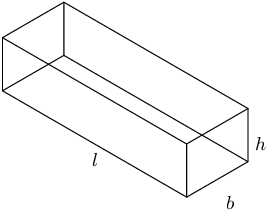$$\begin{array}{rl} \text{Volume}& =\text{area of base}\times \text{height} \\ & =\text{area of rectangle}\times \text{height} \\ & =l\times b\times h \end{array}$$ Triangular prism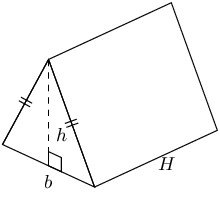$$\begin{array}{rl} \text{Volume}& =\text{area of base}\times \text{height} \\ & =\text{area of triangle}\times \text{height} \\ & =\left(\frac{1}{2}b\times h\right)\times H \end{array}$$ Cylinder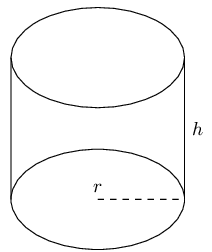$$\begin{array}{rl} \text{Volume}& =\text{area of base}\times \text{height} \\ & =\text{area of circle}\times \text{height} \\ & =\pi {r}^{2}\times h \end{array}$$

## Worked example 5: Finding the volume of a cube

Find the volume of the following cube: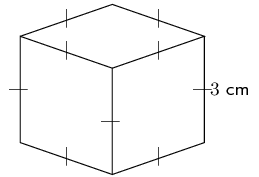### Find the area of the base

\begin{align*} \text{area of square} & = {s}^{2} \\ & = {3}^{2} \\ & = \text{9}\text{ cm$^{2}$} \end{align*}

### Multiply the area of the base by the height of the solid to find the volume

\begin{align*} \text{volume} & = \text{area of base}\times \text{height} \\ & = 9\times 3 \\ & = \text{27}\text{ cm$^{3}$} \end{align*}

The volume of the cube is $$\text{27}$$ $$\text{cm^{3}}$$.

temp text

## Worked example 6: Finding the volume of a triangular prism

Find the volume of the triangular prism: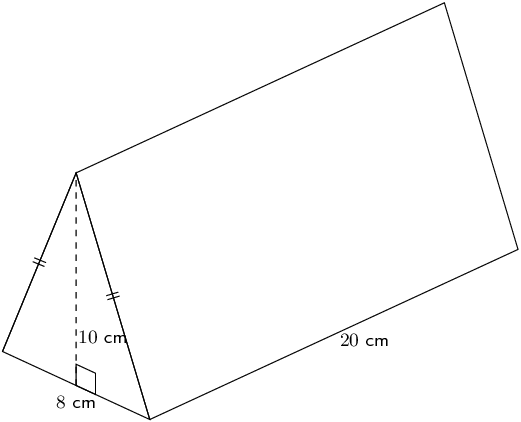### Find the area of the base

\begin{align*} \text{area of triangle} & = \frac{1}{2}b\times h \\ & = \left(\frac{1}{2}\times 8\right)\times 10 \\ & = \text{40}\text{ cm$^{2}$} \end{align*}

### Multiply the area of the base by the height of the solid to find the volume

\begin{align*} \text{volume}& =\text{area of base}\times \text{height} \\ & = \frac{1}{2}b\times h\times H \\ & = 40\times 20 \\ & = \text{800}\text{ cm$^{3}$} \end{align*}

The volume of the triangular prism is $$\text{800}$$ $$\text{cm^{3}}$$.

temp text

## Worked example 7: Finding the volume of a cylinder

Find the volume of the following cylinder (correct to 1 decimal place):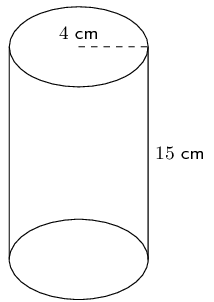### Find the area of the base

\begin{align*} \text{area of circle}& =\pi {r}^{2} \\ & =\pi {\left(4\right)}^{2} \\ & = 16\pi ~\text{cm$^{2}$} \end{align*}

### Multiply the area of the base by the height of the solid to find the volume

\begin{align*} \text{volume}& =\text{area of base}\times \text{height} \\ & =\pi {r}^{2}\times h \\ & =16\pi \times 15 \\ & \approx \text{754,0}\text{ cm$^{3}$} \end{align*}

The volume of the cylinder is $$\text{754,0}$$ $$\text{cm^{3}}$$.

temp text
Textbook Exercise 13.3

Calculate the volumes of the following prisms (correct to $$\text{1}$$ decimal place):\begin{align*} V &= l \times b \times h \\ &= 6 \times 7 \times 10 \\ &= \text{420}\text{ cm$^{3}$} \end{align*}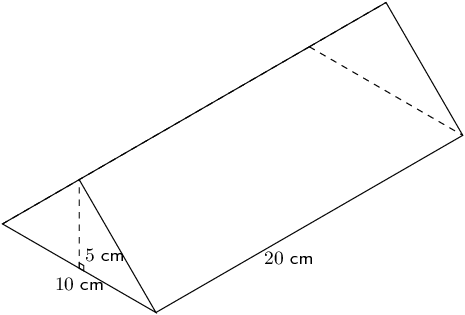\begin{align*} V & = \frac{1}{2} \times b \times h \times H\\ & = \frac{1}{2} \times 10 \times 5 \times 20 \\ & = \text{500}\text{ cm$^{3}$} \end{align*}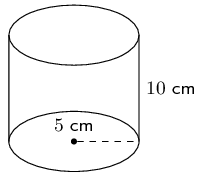\begin{align*} V & = \pi r^2 h \\ & = \pi(5)^2(10)\\ & = 250 \pi\\ & \approx \text{785,4}\text{ cm$^{3}$} \end{align*}

The figure here is a triangular prism. The height of the prism is $$\text{7}$$ units; the triangles, which both contain right angles, have sides which are $$\text{2}$$, $$\sqrt{21}$$ and $$\text{5}$$ units long. Calculate the volume of the figure. Round to two decimal places if necessary.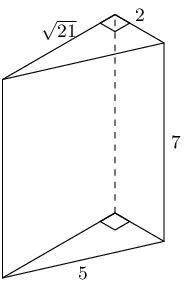\begin{align*} V & = \text{area of base} \times \text{height} \\ & = \left[\frac{1}{2}b_{\triangle}h_{\triangle} \right] (H) \\ & = \left[\frac{1}{2}(\text{2})(\sqrt{21}) \right](\text{7}) \\ & = (\sqrt{21})(\text{7}) \\ & \approx \text{32,06} \end{align*}

The figure here is a rectangular prism. The height of the prism is $$\text{12}$$ units; the other dimensions of the prism are $$\text{11}$$ and $$\text{8}$$ units. Find the volume of the figure.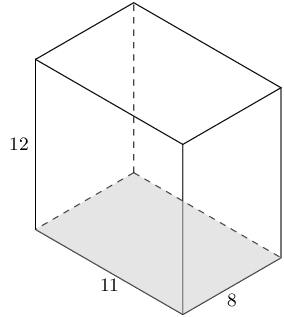\begin{align*} V_{\text{rectangular prism}} & = \text{area of base} \times \text{height} \\ & = (bh)(H) \\ & = (\text{8} \times \text{11})(\text{12}) \\ & = \text{1 056} \end{align*}

The picture below shows a cylinder. The height of the cylinder is $$\text{11}$$ units; the radius of the cylinder is $$r = \text{4}$$ units. Determine the volume of the figure. Round your answer to two decimal places.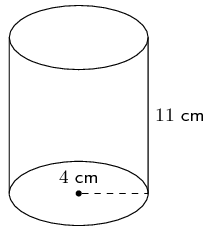\begin{align*} V_{cylinder} & = (\text{area of circle})(H) \\ & = \left[\pi r^{2}\right](H) \\ & = \left[\pi (\text{4})^{2}\right](\text{11}) \\ & = \left[\text{16}\pi\right](\text{11}) \\ & = 176 \pi \\ & \approx \text{552,92} \end{align*}